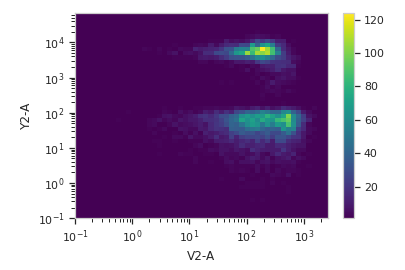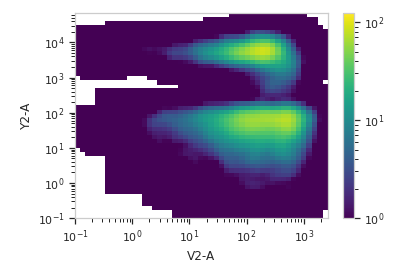# cytoflow.views.densityplot¶

class cytoflow.views.densityplot.DensityView[source]

Plots a 2-d density plot.

huefacet

You must leave the hue facet unset!

Type: None
xchannel, ychannel

The channels to view

Type: Str
xscale, yscale

The scales applied to the data before plotting it.

Type: {‘linear’, ‘log’, ‘logicle’} (default = ‘linear’)
xlim, ylim

Set the min and max limits of the plots’ x and y axes.

Type: (float, float)
xfacet, yfacet

Set to one of the conditions in the Experiment, and a new row or column of subplots will be added for every unique value of that condition.

Type: String
huescale

How should the color scale for huefacet be scaled?

Type: {‘linear’, ‘log’, ‘logicle’}

Examples

Make a little data set.

>>> import cytoflow as flow
>>> import_op = flow.ImportOp()
>>> import_op.tubes = [flow.Tube(file = "Plate01/RFP_Well_A3.fcs",
...                              conditions = {'Dox' : 10.0}),
...                    flow.Tube(file = "Plate01/CFP_Well_A4.fcs",
...                              conditions = {'Dox' : 1.0})]
>>> import_op.conditions = {'Dox' : 'float'}
>>> ex = import_op.apply()


Plot a density plot

>>> flow.DensityView(xchannel = 'V2-A',
...                 xscale = 'log',
...                 ychannel = 'Y2-A',
...                 yscale = 'log').plot(ex)The same plot, smoothed, with a log color scale. Note - you can change the hue scale, even if you don’t have control over the hue facet!

>>> flow.DensityView(xchannel = 'V2-A',
...                  xscale = 'log',
...                  ychannel = 'Y2-A',
...                  yscale = 'log',
...                  huescale = 'log').plot(ex, smoothed = True)plot(experiment, **kwargs)[source]

Plot a faceted density plot view of a channel

Parameters: experiment (Experiment) – The Experiment to plot using this view. title (str) – Set the plot title xlabel, ylabel (str) – Set the X and Y axis labels huelabel (str) – Set the label for the hue facet (in the legend) legend (bool) – Plot a legend for the color or hue facet? Defaults to True. sharex, sharey (bool) – If there are multiple subplots, should they share axes? Defaults to True. row_order, col_order, hue_order (list) – Override the row/column/hue facet value order with the given list. If a value is not given in the ordering, it is not plotted. Defaults to a “natural ordering” of all the values. height (float) – The height of each row in inches. Default = 3.0 aspect (float) – The aspect ratio of each subplot. Default = 1.5 col_wrap (int) – If xfacet is set and yfacet is not set, you can “wrap” the subplots around so that they form a multi-row grid by setting col_wrap to the number of columns you want. sns_style ({“darkgrid”, “whitegrid”, “dark”, “white”, “ticks”}) – Which seaborn style to apply to the plot? Default is whitegrid. sns_context ({“paper”, “notebook”, “talk”, “poster”}) – Which seaborn context to use? Controls the scaling of plot elements such as tick labels and the legend. Default is talk. despine (Bool) – Remove the top and right axes from the plot? Default is True. min_quantile (float (>0.0 and <1.0, default = 0.001)) – Clip data that is less than this quantile. max_quantile (float (>0.0 and <1.0, default = 1.00)) – Clip data that is greater than this quantile. xlim, ylim ((float, float)) – Set the range of the plot’s axis. gridsize (int) – The size of the grid on each axis. Default = 50 smoothed (bool) – Should the resulting mesh be smoothed? smoothed_sigma (int) – The standard deviation of the smoothing kernel. default = 1. cmap (cmap) – An instance of matplotlib.colors.Colormap. By default, the viridis colormap is used under_color (matplotlib color) – Sets the color to be used for low out-of-range values. bad_color (matplotlib color) – Set the color to be used for masked values.

Notes

Other kwargs are passed to matplotlib.axes.Axes.pcolormesh